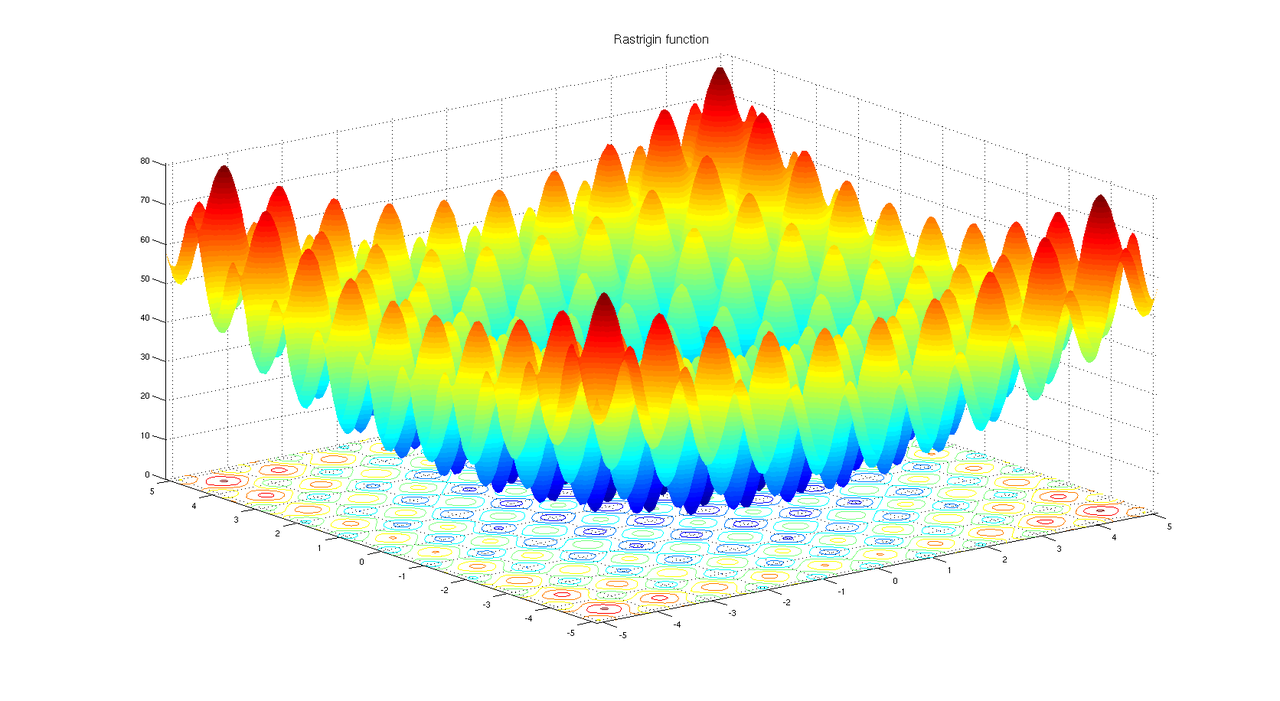{{ message }}

# Find the global minimum of Rastring functionIn mathematical optimization, the Rastrigin function is a non-convex function used as a performance test problem for optimization algorithms. It's a typical example of non-linear multimodal function. It was first proposed by Rastrigin (Systems of extremal control Mir, Moscow 1974) as a 2-dimensional function and has been generalized by Mühlenbein, Schomisch and Born (The Parallel Genetic Algorithm as Function Optimizer, Parallel Computing 17, 1991).

Finding the minimum of this function is a fairly difficult problem due to its large search space and its large number of local minima.

On an n-dimensional domain it's defined by:where A=10. The function is usually evaluated on the hypercube xᵢ ∈ [-5.12, 5.12].

As the plot shows, Rastrigin's function has many local minima. However there is just one global minimum which occurs at x = 0, where the value of the function is 0. At any local minimum other than 0, the value of Rastrigin's function is greater than 0. The farther the local minimum is from the origin, the larger the value of the function is at that point.

Rastrigin's function is often used for testing, because its many local minima make it difficult for standard, gradient-based methods to find the global minimum.

We will use Differential Evolution to find out the global minimum of the five-dimensional Rastrigin function.

The function can be written as:

double neg_rastrigin(const std::vector<double> &x)
{
constexpr double  A = 10.0;
constexpr double PI =  3.141592653589793;

const double rastrigin =
A * x.size() + std::accumulate(x.begin(), x.end(), 0.0,
[](double sum, double xi)
{
return sum + xi*xi - A*std::cos(2*PI*xi);
});

return -rastrigin;
}

Observe how we have defined the negation of the Rastrigin function. Vita always tries to maximize a function, if you want the minimum of a function you must consider its negation (maximizing a function is equivalent to minimizing its negative).

We specify the search space:

const unsigned dimensions(5);  // 5D - Rastrigin function

vita::de_problem prob(dimensions, {-5.12, 5.12});

and some of the Differential Evolution parameters:

prob.env.individuals =   50;
prob.env.generations = 1000;

The vita::de_search class constructor links the search space to the objective function and the de_search::run member function starts the search:

vita::de_search<decltype(neg_rastrigin)> search(prob, neg_rastrigin);

const auto res(search.run());

The default is to perform a single evolutionary run. EAs are stochastic processes, there is no guarantee they will discover the global optimum. In general it's a good idea to perform multiple runs (search.run(n)).

The remaining code just prints the candidate solution:

const auto solution(  res.best.solution);
const auto value(res.best.score.fitness);

std::cout << "Minimum: " <<  value << '\n';

std::cout << "Coordinates: [ ";
for (auto xi : solution)
std::cout << xi << ' ';
std::cout << "]\n";

Differential Evolution is a general purpose, "black box" optimization algorithm so you can easily adapt this example to different functions.

The complete source code of the example (also available here):

#include "kernel/vita.h"

double neg_rastrigin(const std::vector<double> &x)
{
constexpr double  A = 10.0;
constexpr double PI =  3.141592653589793;

const double rastrigin =
A * x.size() + std::accumulate(x.begin(), x.end(), 0.0,
[](double sum, double xi)
{
return sum + xi*xi - A*std::cos(2*PI*xi);
});

return -rastrigin;
}

int main()
{
const unsigned dimensions(5);  // 5D - Rastrigin function

vita::de_problem prob(dimensions, {-5.12, 5.12});

prob.env.individuals =   50;
prob.env.generations = 1000;

vita::de_search<decltype(neg_rastrigin)> search(prob, neg_rastrigin);

const auto res(search.run());

const auto solution(  res.best.solution);
const auto value(res.best.score.fitness);

std::cout << "Minimum found: " <<  value << '\n';

std::cout << "Coordinates: [ ";
for (auto xi : solution)
std::cout << xi << ' ';
std::cout << "]\n";
}